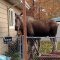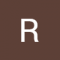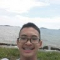Class Central is learner-supported. When you buy through links on our site, we may earn an affiliate commission.

# Data Science Math Skills

### Overview

##### Class Central Tips
Data science courses contain math—no avoiding that! This course is designed to teach learners the basic math you will need in order to be successful in almost any data science math course and was created for learners who have basic math skills but may not have taken algebra or pre-calculus. Data Science Math Skills introduces the core math that data science is built upon, with no extra complexity, introducing unfamiliar ideas and math symbols one-at-a-time.

Learners who complete this course will master the vocabulary, notation, concepts, and algebra rules that all data scientists must know before moving on to more advanced material.

Topics include:
~Set theory, including Venn diagrams
~Properties of the real number line
~Interval notation and algebra with inequalities
~Uses for summation and Sigma notation
~Math on the Cartesian (x,y) plane, slope and distance formulas
~Graphing and describing functions and their inverses on the x-y plane,
~The concept of instantaneous rate of change and tangent lines to a curve
~Exponents, logarithms, and the natural log function.
~Probability theory, including Bayes’ theorem.

While this course is intended as a general introduction to the math skills needed for data science, it can be considered a prerequisite for learners interested in the course, "Mastering Data Analysis in Excel," which is part of the Excel to MySQL Data Science Specialization. Learners who master Data Science Math Skills will be fully prepared for success with the more advanced math concepts introduced in "Mastering Data Analysis in Excel."

Good luck and we hope you enjoy the course!

### Syllabus

• Welcome to Data Science Math Skills
• This short module includes an overview of the course's structure, working process, and information about course certificates, quizzes, video lectures, and other important course details. Make sure to read it right away and refer back to it whenever needed
• Building Blocks for Problem Solving
• This module contains three lessons that are build to basic math vocabulary. The first lesson, "Sets and What They’re Good For," walks you through the basic notions of set theory, including unions, intersections, and cardinality. It also gives a real-world application to medical testing. The second lesson, "The Infinite World of Real Numbers," explains notation we use to discuss intervals on the real number line. The module concludes with the third lesson, "That Jagged S Symbol," where you will learn how to compactly express a long series of additions and use this skill to define statistical quantities like mean and variance.
• Functions and Graphs
• This module builds vocabulary for graphing functions in the plane. In the first lesson, "Descartes Was Really Smart," you will get to know the Cartesian Plane, measure distance in it, and find the equations of lines. The second lesson introduces the idea of a function as an input-output machine, shows you how to graph functions in the Cartesian Plane, and goes over important vocabulary.
• Measuring Rates of Change
• This module begins a very gentle introduction to the calculus concept of the derivative. The first lesson, "This is About the Derivative Stuff," will give basic definitions, work a few examples, and show you how to apply these concepts to the real-world problem of optimization. We then turn to exponents and logarithms, and explain the rules and notation for these math tools. Finally we learn about the rate of change of continuous growth, and the special constant known as “e” that captures this concept in a single number—near 2.718.
• Introduction to Probability Theory
• This module introduces the vocabulary and notation of probability theory – mathematics for the study of outcomes that are uncertain but have predictable rates of occurrence.

We start with the basic definitions and rules of probability, including the probability of two or more events both occurring, the sum rule and the product rule, and then proceed to Bayes’ Theorem and how it is used in practical problems.

## Reviews

4.1 rating, based on 12 Class Central reviews

4.5 rating at Coursera based on 11324 ratings

Start your review of Data Science Math Skills

• Benjamin Phua completed this course, spending 15 hours a week on it and found the course difficulty to be medium.

Summary of Course Review: I was fairly satisfied with the course as an introduction to the math for data science specifically and given there aren't many math courses built specifically for an introduction to Data Science provided by a credible Uni…
• The course was good, but it was too simple for me. This may be a problem with the labeling. When I saw Data Science in the title, I expected somewhat more advanced topics.
The last section on Bayes Theorem was useful though. Especially the last part on calculation of prior versus posterior probabilities.
If you are not knowledgeable about the more basic concepts of logarithms, exponents, etc., or need to brush up on them in a short period of time, you would find the course beneficial in my opinion.
• Mani Kumar Reddy Kancharla completed this course, spending 1 hours a week on it and found the course difficulty to be very easy.

It was easy, being a graduate myself, the course was very easy, it was more of brushing up my math knowledge I learnt in High School. It was very interactive and very well explained.

Although there were a lot of mistakes in the course, everything has been corrected with right measures.

I strongly recommend this course for anyone who wants to brush up their math skills.
•Míša Charvátová

Míša Charvátová completed this course, spending 2 hours a week on it and found the course difficulty to be medium.

The beginning of the course was great, the first teacher explained everything slowly and thoroughly - even trivial basics, but it seems necessary in this kind of the course.
The second teacher on the other hand was explaining thing in complicated way assuming you understand everything at first sight, juggeling quickly with formulas and not explaining the background of what is happening in the formula.
• Relatively interesting, however not complex enough. It also covers only selected, introductory topics, far from all the math needed for making actually "data science".
Still – worth making. Good educational materials and exercises.
• Anonymous
Data science courses contain math—no avoiding that! This course is designed to teach learners the basic math you will need in order to be successful in almost any data science math course and was created for learners who have basic math skills but may not have taken algebra or pre-calculus. Data Science Math Skills introduces the core math that data science is built upon, with no extra complexity, introducing unfamiliar ideas and math symbols one-at-a-time.
• M SURESH BABU

M SURESH BABU is taking this course right now, spending 4 hours a week on it and found the course difficulty to be very easy.

The key to solving simple algebraic equations containing a single unknown (e.g. x + 6 = 10) is to realize that the equation is an equality. As long as you do the same mathematical operation (e.g. add a constant, subtract a constant, multiply by a constant, and divide by a constant) to both sides of the equation, the equality is still an equality. This includes squaring both sides of the equation or taking the square root of both sides of the equation.
• Anonymous

Anonymous completed this course.

The lecturer I think is very nice to listen to. The concepts are pretty simple but they go over the notation pretty well. I think it's a pretty good way to get your math foundations ready for data science. Week 4 was definitely pretty confusing, but overall the course is quite doable and definitely useful for those going into data science who aren't strong in math.
•Rupkumar Sarkar

Rupkumar Sarkar is taking this course right now.

I will finsh tyis course in 3 Months.This course isvery interesting to me .i love this couse apart from thatvi will do hard work with my best of .I really greatfull to coursesera platfrokm to giveeva big opportunity to learn this couse.thankvu to coursesera platfrom to provide this course
• Kondaamitha

Kondaamitha is taking this course right now, spending 6 hours a week on it and found the course difficulty to be medium.

I think it is very helpful so iam much interested in this course as a cse student and one more thing I want a non credit certificate so kindly provide this opportunity
• Burra Venkata Sesha Talpa Sai is taking this course right now, spending 20 hours a week on it and found the course difficulty to be medium.

As I am a beginner for this course I will follow the guidelines of course designer. I with mathematics back ground and some basic knowledge of computer Science
•NAPHAT PERMPREDANUN

NAPHAT PERMPREDANUN completed this course, spending 4 hours a week on it and found the course difficulty to be easy.

I think this course provide a great amount of Data science for the starters to study. Since in the higher level there will be a problem more with the function distribution, the normal distribution on statistics, and so on, studying some basics beforehand makes a better understanding on them.

### Never Stop Learning.

Get personalized course recommendations, track subjects and courses with reminders, and more.